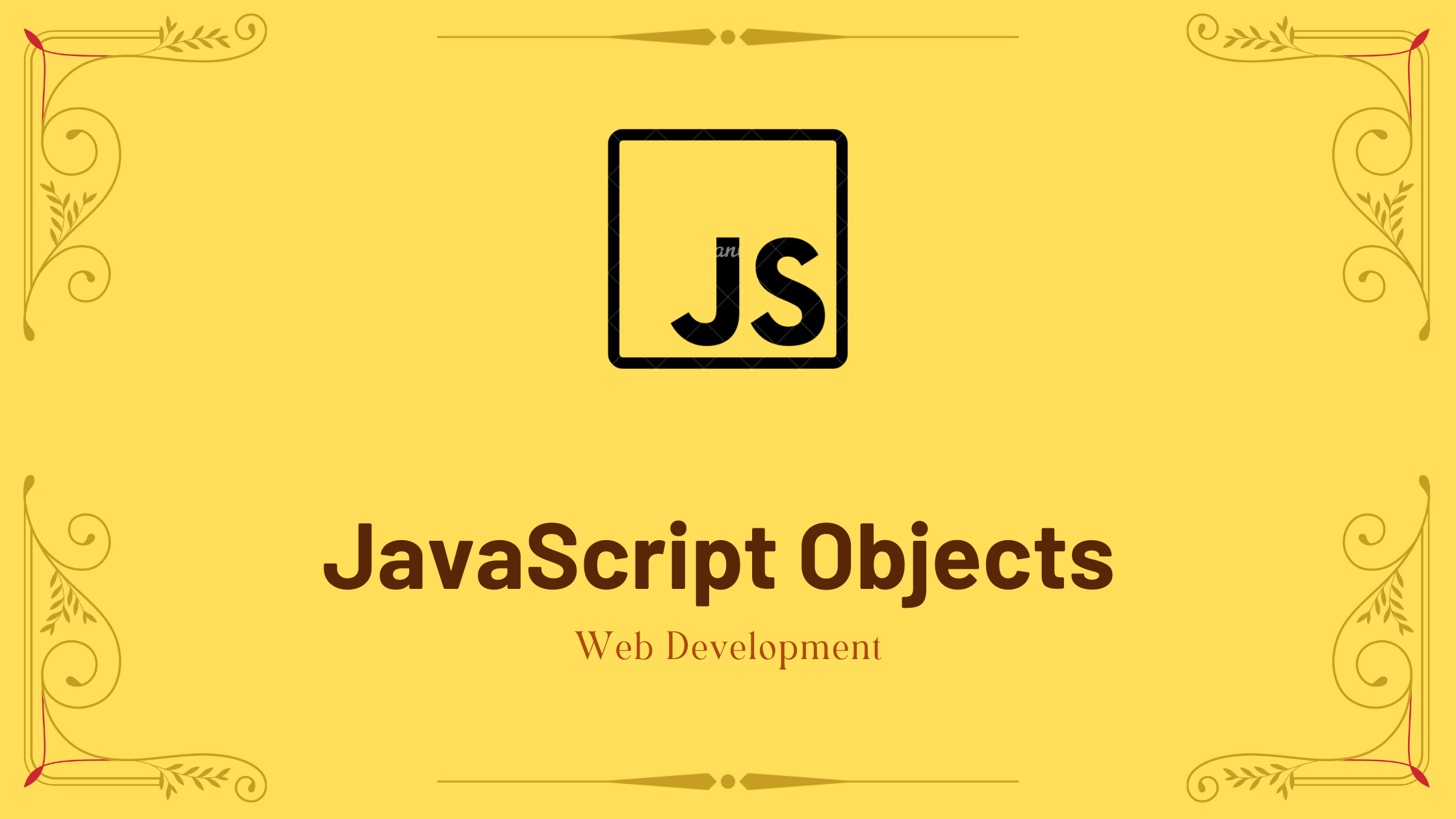## JavaScript Objects With Example

In this tutorial, you will learn about JavaScript object with the help of examples.

You learned about seven different primitive data types in the JavaScript data types tutorial. And now you’ll learn about the eighth data type (JavaScript object).

A non-primitive data type that allows you to store several collections of data is a JavaScript object.

Note: Javascript objects differ slightly from those used in other programming languages. Creating objects does not necessitate the creation of classes.

An example of a JavaScript object is shown below.

```// object
const student = {
firstName: 'ram',
class: 10
};```

In this case, a student is an object that stores things like strings and numbers.

Contents

## JavaScript Object Declaration

The syntax for declaring an object is as follows:

```const object_name = {
key1: value1,
key2: value2
}```

An object named object name is defined here. Every component of an object is a key: a value pair separated by commas and surrounded by curly braces{}.

For example:

```// object creation
const person = {
name: 'John',
age: 20
};
console.log(typeof person); // object```

An object can also be defined in a single line.

const person = { name: ‘John’, age: 20 };

In the preceding example, the keys are name and age, and the values are John and 20.

## JavaScript Object Properties

Properties are what “key: value” pairs are known as in JavaScript. For instance:

```let person = {
name: 'John',
age: 20
};```

Name: “John” and age: 20 are properties in this case.

## Accessing Object Properties

The key to a property can be used to gain access to its value.

1. Using dot Notation

The dot notation’s syntax is shown here.

objectName.key

For example:

```const person = {
name: 'John',
age: 20,
};

// accessing property
console.log(person.name); // John```

2. Using bracket Notation

Here is the syntax of the bracket notation.

`objectName["propertyName"]`

For example:

```const person = {
name: 'John',
age: 20,
};

// accessing property
console.log(person["name"]); // John```

## JavaScript Nested Objects

Another object may be contained within an object. For instance:

```// nested object
const student = {
name: 'John',
age: 20,
marks: {
science: 70,
math: 75
}
}

// accessing property of student object
console.log(student.marks); // {science: 70, math: 75}

// accessing property of marks object
console.log(student.marks.science); // 70```

An object student in the aforementioned example has an object value in the marks property.

## JavaScript Object Methods

In JavaScript, a function can also be contained in an objects.

```const person = {
name: 'Sam',
age: 30,
// using function as a value
greet: function() { console.log('hello') }
}

person.greet(); // hello```

In this case, the greet key’s value is a function. We must use somebody because of this. instead of a person, use greet(). to invoke the object’s internal function.

You may like:

Switch Statement in JavaScript